Скачать презентацию NMIMS PTMBA FIN DIV A B ARBITRAGE PRICING

59c13b3f20bb2bf37c7940c9c8080b17.ppt

• Количество слайдов: 32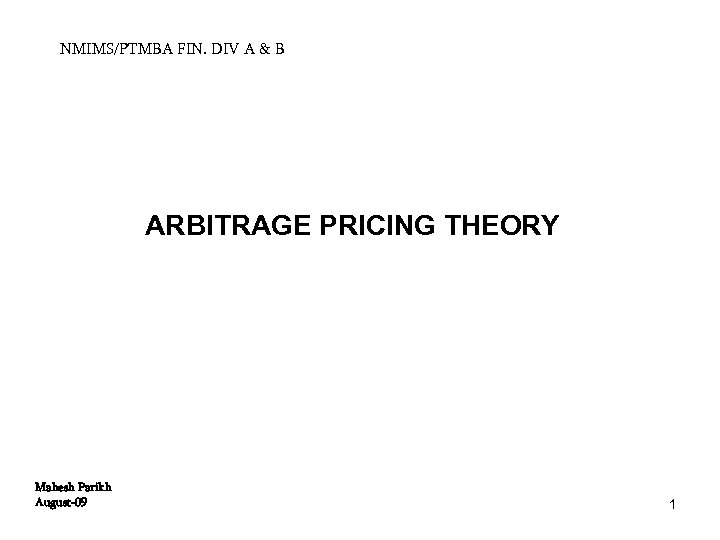NMIMS/PTMBA FIN. DIV A & B ARBITRAGE PRICING THEORY Mahesh Parikh August-09 1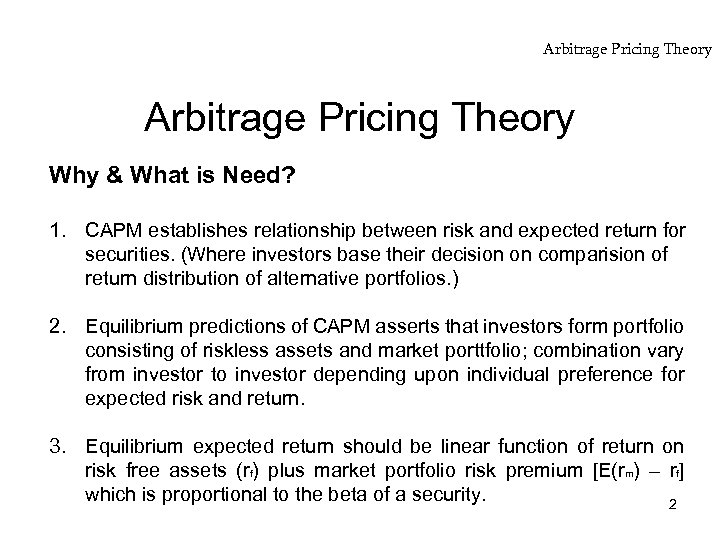Arbitrage Pricing Theory Why & What is Need? 1. CAPM establishes relationship between risk and expected return for securities. (Where investors base their decision on comparision of return distribution of alternative portfolios. ) 2. Equilibrium predictions of CAPM asserts that investors form portfolio consisting of riskless assets and market porttfolio; combination vary from investor to investor depending upon individual preference for expected risk and return. 3. Equilibrium expected return should be linear function of return on risk free assets (rf) plus market portfolio risk premium [E(rm) – rf] which is proportional to the beta of a security. 2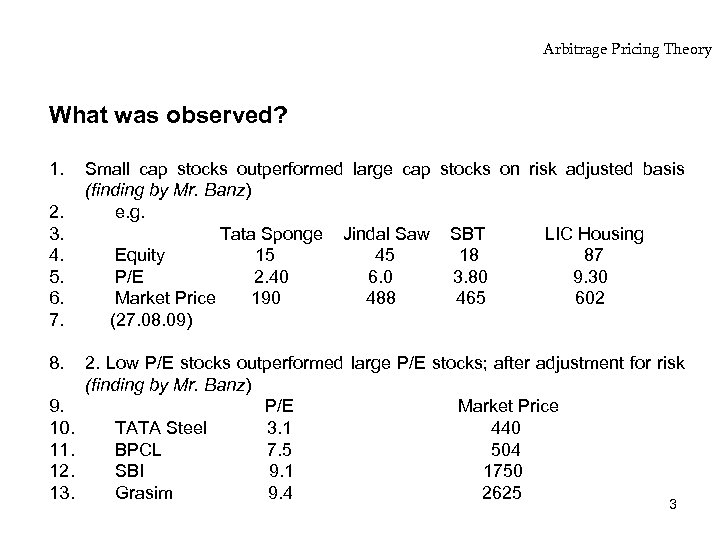Arbitrage Pricing Theory What was observed? 1. 2. 3. 4. 5. 6. 7. 8. 9. 10. 11. 12. 13. Small cap stocks outperformed large cap stocks on risk adjusted basis (finding by Mr. Banz) e. g. Tata Sponge Jindal Saw SBT LIC Housing Equity 15 45 18 87 P/E 2. 40 6. 0 3. 80 9. 30 Market Price 190 488 465 602 (27. 08. 09) 2. Low P/E stocks outperformed large P/E stocks; after adjustment for risk (finding by Mr. Banz) P/E Market Price TATA Steel 3. 1 440 BPCL 7. 5 504 SBI 9. 1 1750 Grasim 9. 4 2625 3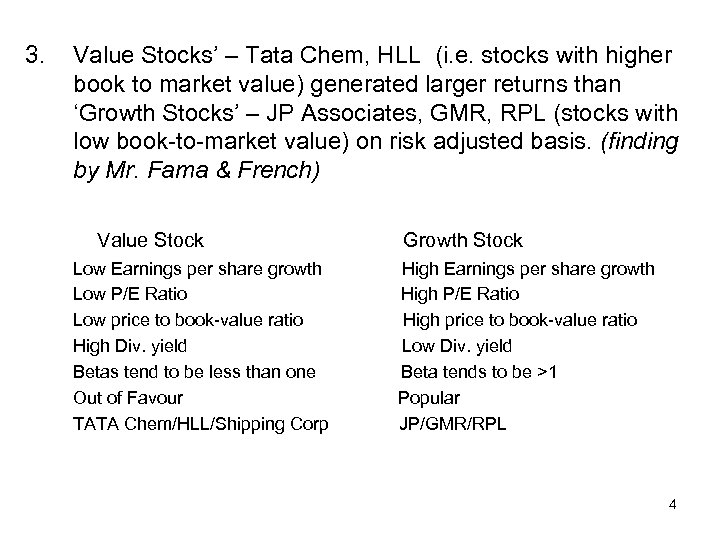3. Value Stocks’ – Tata Chem, HLL (i. e. stocks with higher book to market value) generated larger returns than ‘Growth Stocks’ – JP Associates, GMR, RPL (stocks with low book-to-market value) on risk adjusted basis. (finding by Mr. Fama & French) Value Stock Low Earnings per share growth Low P/E Ratio Low price to book-value ratio High Div. yield Betas tend to be less than one Out of Favour TATA Chem/HLL/Shipping Corp Growth Stock High Earnings per share growth High P/E Ratio High price to book-value ratio Low Div. yield Beta tends to be >1 Popular JP/GMR/RPL 4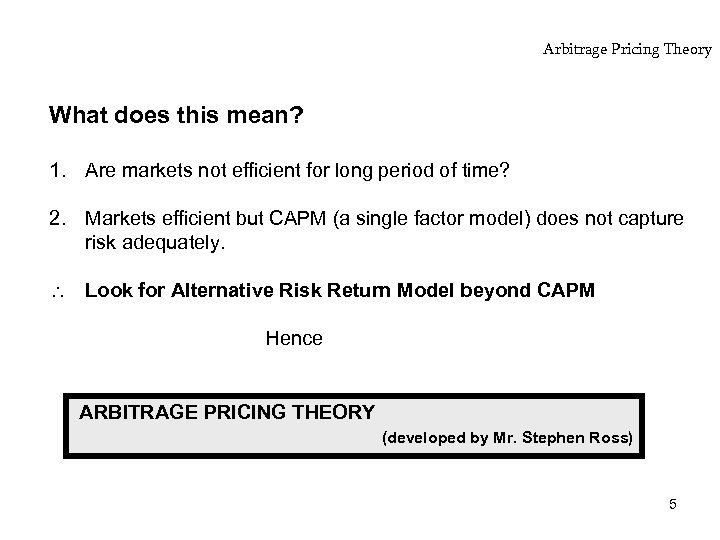Arbitrage Pricing Theory What does this mean? 1. Are markets not efficient for long period of time? 2. Markets efficient but CAPM (a single factor model) does not capture risk adequately. Look for Alternative Risk Return Model beyond CAPM Hence ARBITRAGE PRICING THEORY (developed by Mr. Stephen Ross) 5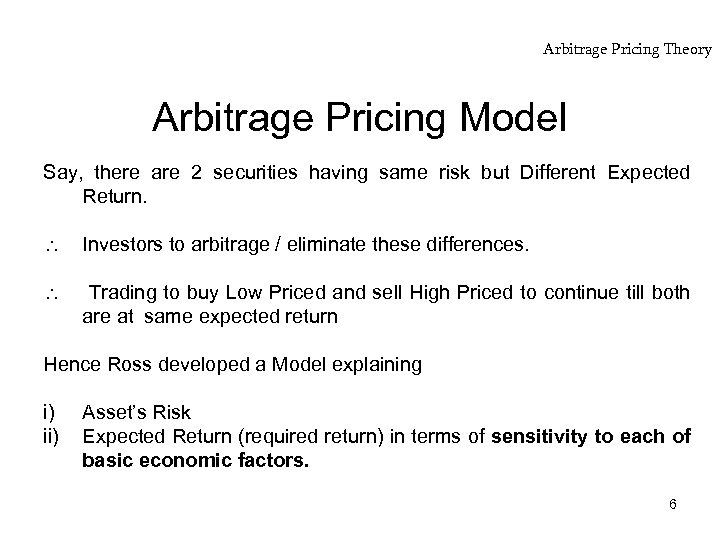Arbitrage Pricing Theory Arbitrage Pricing Model Say, there are 2 securities having same risk but Different Expected Return. Investors to arbitrage / eliminate these differences. Trading to buy Low Priced and sell High Priced to continue till both are at same expected return Hence Ross developed a Model explaining i) ii) Asset’s Risk Expected Return (required return) in terms of sensitivity to each of basic economic factors. 6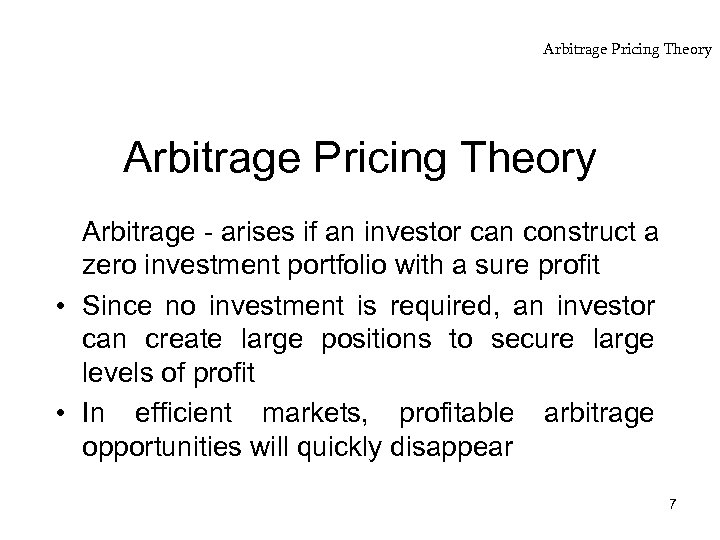Arbitrage Pricing Theory Arbitrage - arises if an investor can construct a zero investment portfolio with a sure profit • Since no investment is required, an investor can create large positions to secure large levels of profit • In efficient markets, profitable arbitrage opportunities will quickly disappear 7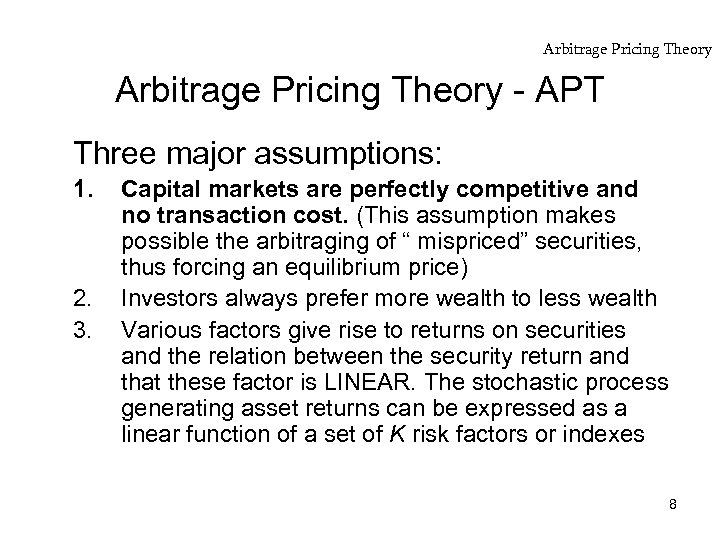Arbitrage Pricing Theory - APT Three major assumptions: 1. 2. 3. Capital markets are perfectly competitive and no transaction cost. (This assumption makes possible the arbitraging of “ mispriced” securities, thus forcing an equilibrium price) Investors always prefer more wealth to less wealth Various factors give rise to returns on securities and the relation between the security return and that these factor is LINEAR. The stochastic process generating asset returns can be expressed as a linear function of a set of K risk factors or indexes 8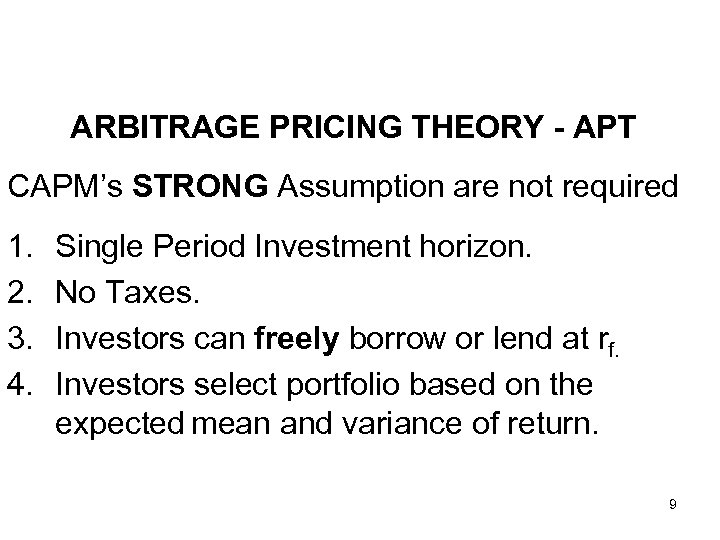ARBITRAGE PRICING THEORY - APT CAPM’s STRONG Assumption are not required 1. 2. 3. 4. Single Period Investment horizon. No Taxes. Investors can freely borrow or lend at rf. Investors select portfolio based on the expected mean and variance of return. 9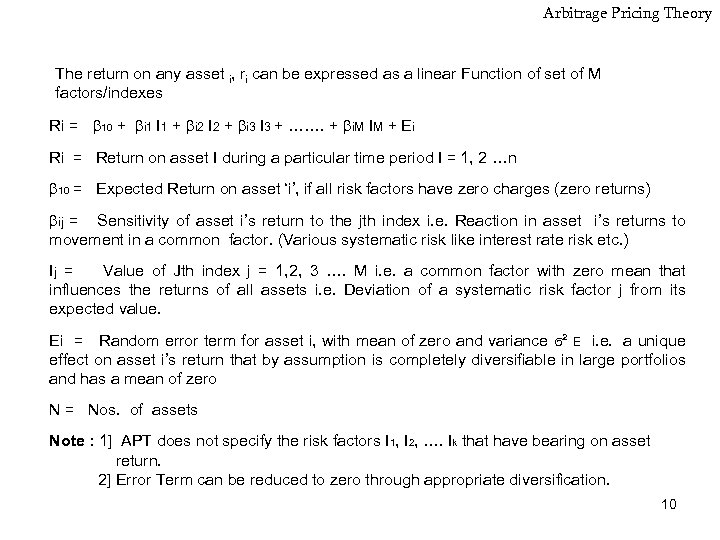Arbitrage Pricing Theory The return on any asset i, ri can be expressed as a linear Function of set of M factors/indexes Ri = 10 + i 1 I 1 + i 2 I 2 + i 3 I 3 + ……. + i. M IM + Ei Ri = Return on asset I during a particular time period I = 1, 2 …n 10 = Expected Return on asset ‘i’, if all risk factors have zero charges (zero returns) ij = Sensitivity of asset i’s return to the jth index i. e. Reaction in asset i’s returns to movement in a common factor. (Various systematic risk like interest rate risk etc. ) Ij = Value of Jth index j = 1, 2, 3 …. M i. e. a common factor with zero mean that influences the returns of all assets i. e. Deviation of a systematic risk factor j from its expected value. Ei = Random error term for asset i, with mean of zero and variance ϭ² E i. e. a unique effect on asset i’s return that by assumption is completely diversifiable in large portfolios and has a mean of zero N = Nos. of assets Note : 1] APT does not specify the risk factors I 1, I 2, …. Ik that have bearing on asset return. 2] Error Term can be reduced to zero through appropriate diversification. 10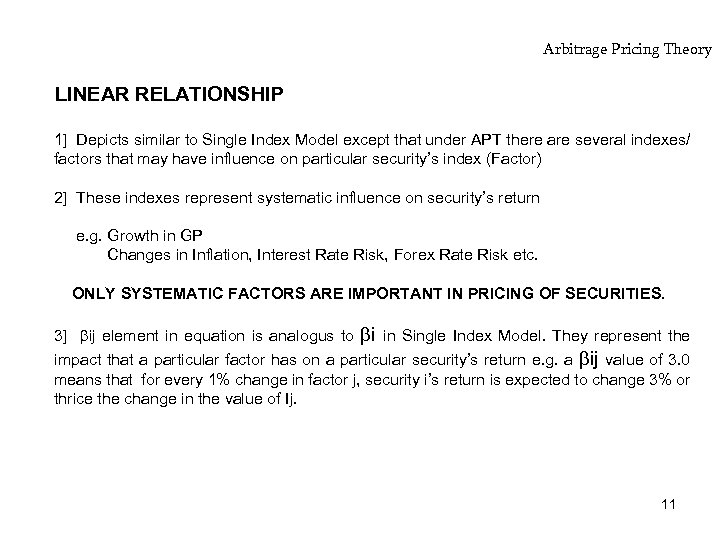Arbitrage Pricing Theory LINEAR RELATIONSHIP 1] Depicts similar to Single Index Model except that under APT there are several indexes/ factors that may have influence on particular security’s index (Factor) 2] These indexes represent systematic influence on security’s return e. g. Growth in GP Changes in Inflation, Interest Rate Risk, Forex Rate Risk etc. ONLY SYSTEMATIC FACTORS ARE IMPORTANT IN PRICING OF SECURITIES. 3] ij element in equation is analogus to i in Single Index Model. They represent the impact that a particular factor has on a particular security’s return e. g. a ij value of 3. 0 means that for every 1% change in factor j, security i’s return is expected to change 3% or thrice the change in the value of Ij. 11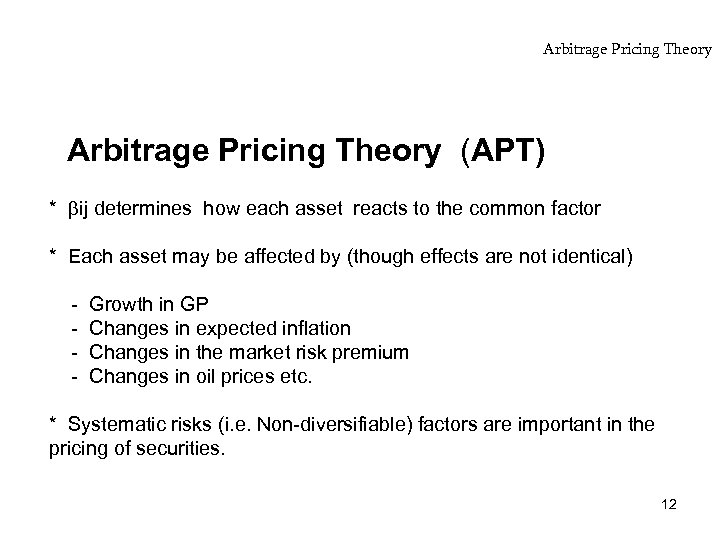Arbitrage Pricing Theory (APT) * ij determines how each asset reacts to the common factor * Each asset may be affected by (though effects are not identical) - Growth in GP Changes in expected inflation Changes in the market risk premium Changes in oil prices etc. * Systematic risks (i. e. Non-diversifiable) factors are important in the pricing of securities. 12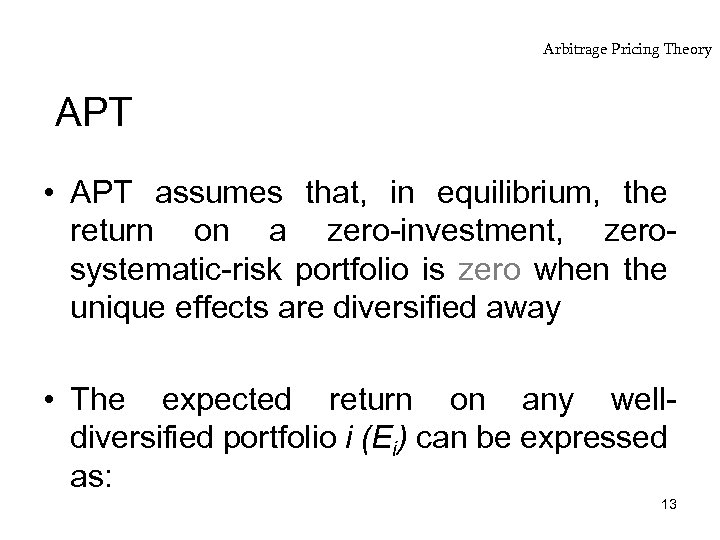Arbitrage Pricing Theory APT • APT assumes that, in equilibrium, the return on a zero-investment, zerosystematic-risk portfolio is zero when the unique effects are diversified away • The expected return on any welldiversified portfolio i (Ei) can be expressed as: 13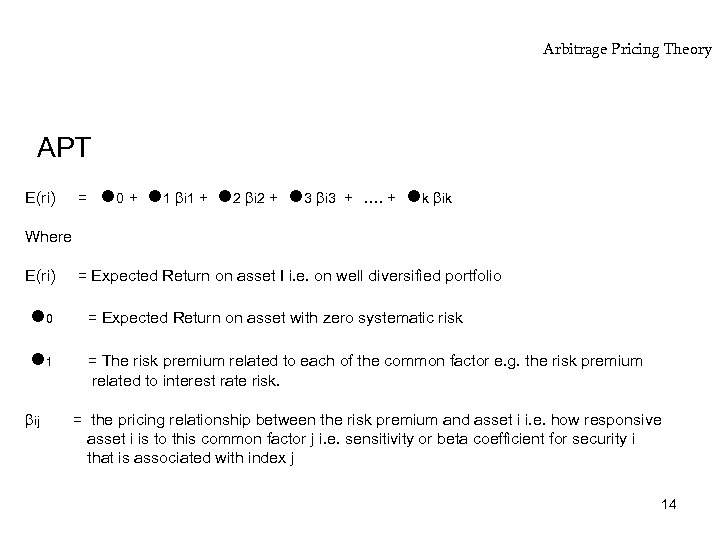Arbitrage Pricing Theory APT E(ri) = 0 + 1 i 1 + 2 i 2 + 3 i 3 + …. + k ik Where E(ri) = Expected Return on asset I i. e. on well diversified portfolio 0 = Expected Return on asset with zero systematic risk 1 = The risk premium related to each of the common factor e. g. the risk premium related to interest rate risk. ij = the pricing relationship between the risk premium and asset i i. e. how responsive asset i is to this common factor j i. e. sensitivity or beta coefficient for security i that is associated with index j 14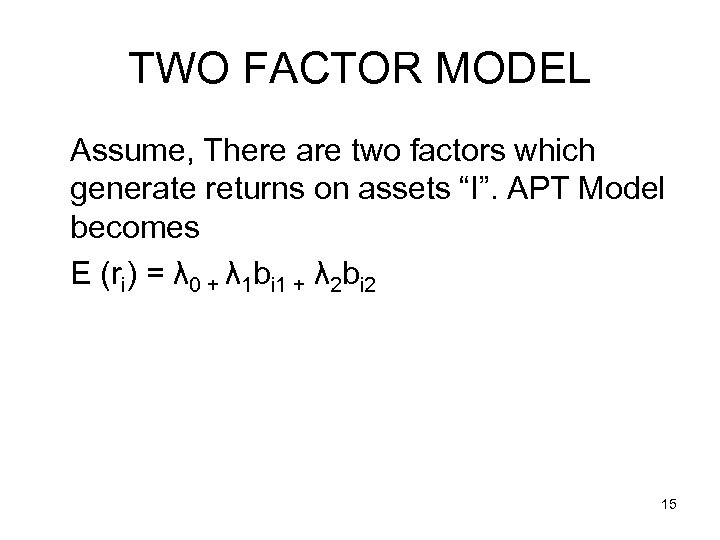TWO FACTOR MODEL Assume, There are two factors which generate returns on assets “I”. APT Model becomes E (ri) = λ 0 + λ 1 bi 1 + λ 2 bi 2 15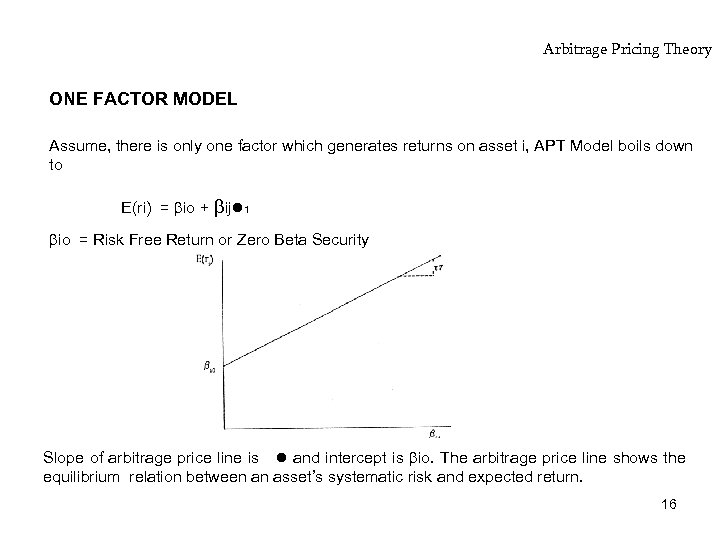Arbitrage Pricing Theory ONE FACTOR MODEL Assume, there is only one factor which generates returns on asset i, APT Model boils down to E(ri) = io + ij 1 io = Risk Free Return or Zero Beta Security Slope of arbitrage price line is and intercept is io. The arbitrage price line shows the equilibrium relation between an asset’s systematic risk and expected return. 16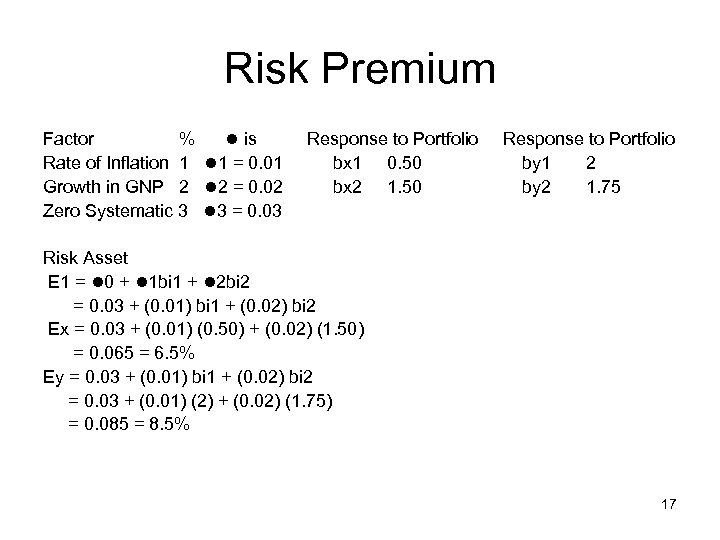Risk Premium Factor % is Rate of Inflation 1 1 = 0. 01 Growth in GNP 2 2 = 0. 02 Zero Systematic 3 3 = 0. 03 Response to Portfolio bx 1 0. 50 bx 2 1. 50 Response to Portfolio by 1 2 by 2 1. 75 Risk Asset E 1 = 0 + 1 bi 1 + 2 bi 2 = 0. 03 + (0. 01) bi 1 + (0. 02) bi 2 Ex = 0. 03 + (0. 01) (0. 50) + (0. 02) (1. 50) = 0. 065 = 6. 5% Ey = 0. 03 + (0. 01) bi 1 + (0. 02) bi 2 = 0. 03 + (0. 01) (2) + (0. 02) (1. 75) = 0. 085 = 8. 5% 17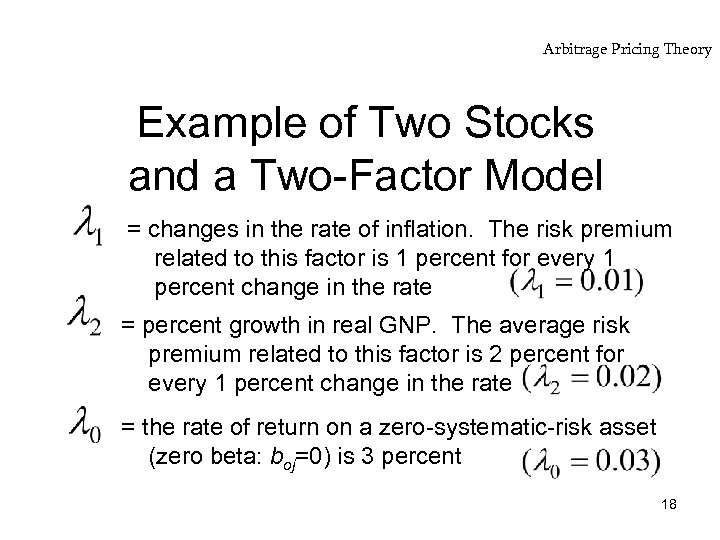Arbitrage Pricing Theory Example of Two Stocks and a Two-Factor Model = changes in the rate of inflation. The risk premium related to this factor is 1 percent for every 1 percent change in the rate = percent growth in real GNP. The average risk premium related to this factor is 2 percent for every 1 percent change in the rate = the rate of return on a zero-systematic-risk asset (zero beta: boj=0) is 3 percent 18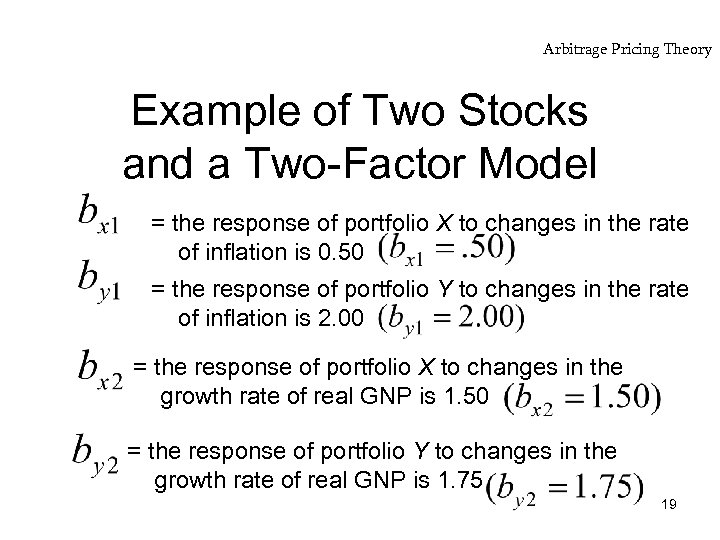Arbitrage Pricing Theory Example of Two Stocks and a Two-Factor Model = the response of portfolio X to changes in the rate of inflation is 0. 50 = the response of portfolio Y to changes in the rate of inflation is 2. 00 = the response of portfolio X to changes in the growth rate of real GNP is 1. 50 = the response of portfolio Y to changes in the growth rate of real GNP is 1. 75 19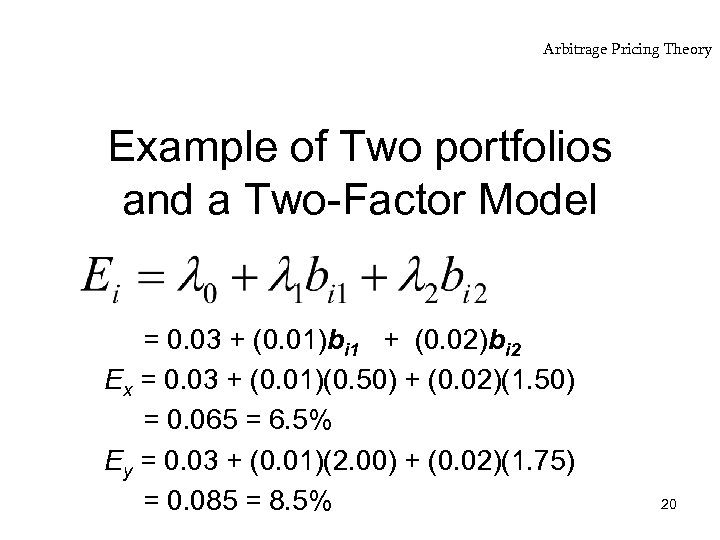Arbitrage Pricing Theory Example of Two portfolios and a Two-Factor Model = 0. 03 + (0. 01)bi 1 + (0. 02)bi 2 Ex = 0. 03 + (0. 01)(0. 50) + (0. 02)(1. 50) = 0. 065 = 6. 5% Ey = 0. 03 + (0. 01)(2. 00) + (0. 02)(1. 75) = 0. 085 = 8. 5% 20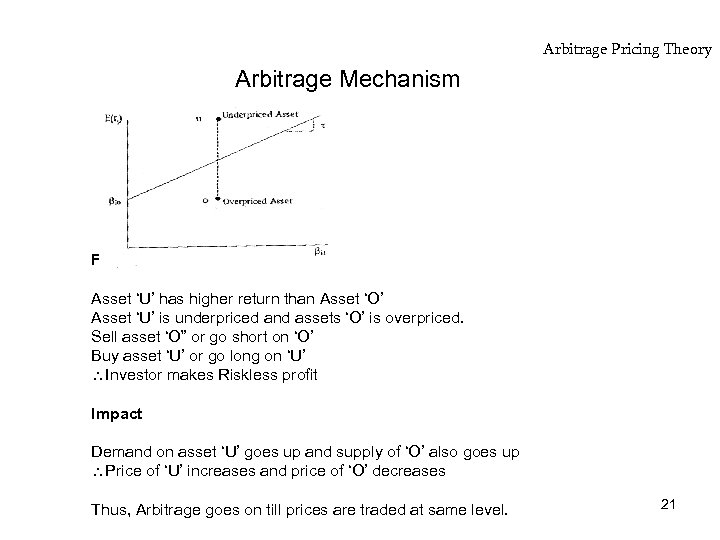Arbitrage Pricing Theory Arbitrage Mechanism For same risks Asset ‘U’ has higher return than Asset ‘O’ Asset ‘U’ is underpriced and assets ‘O’ is overpriced. Sell asset ‘O” or go short on ‘O’ Buy asset ‘U’ or go long on ‘U’ Investor makes Riskless profit Impact Demand on asset ‘U’ goes up and supply of ‘O’ also goes up Price of ‘U’ increases and price of ‘O’ decreases Thus, Arbitrage goes on till prices are traded at same level. 21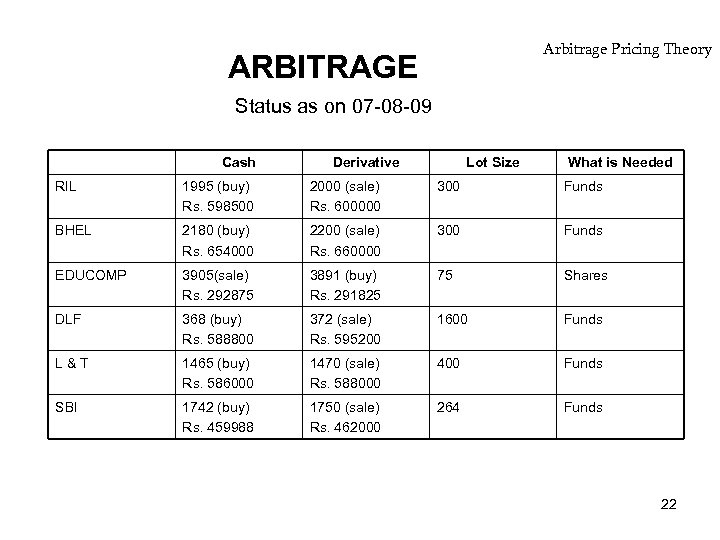Arbitrage Pricing Theory ARBITRAGE Status as on 07 -08 -09 Cash Derivative Lot Size What is Needed RIL 1995 (buy) Rs. 598500 2000 (sale) Rs. 600000 300 Funds BHEL 2180 (buy) Rs. 654000 2200 (sale) Rs. 660000 300 Funds EDUCOMP 3905(sale) Rs. 292875 3891 (buy) Rs. 291825 75 Shares DLF 368 (buy) Rs. 588800 372 (sale) Rs. 595200 1600 Funds L&T 1465 (buy) Rs. 586000 1470 (sale) Rs. 588000 400 Funds SBI 1742 (buy) Rs. 459988 1750 (sale) Rs. 462000 264 Funds 22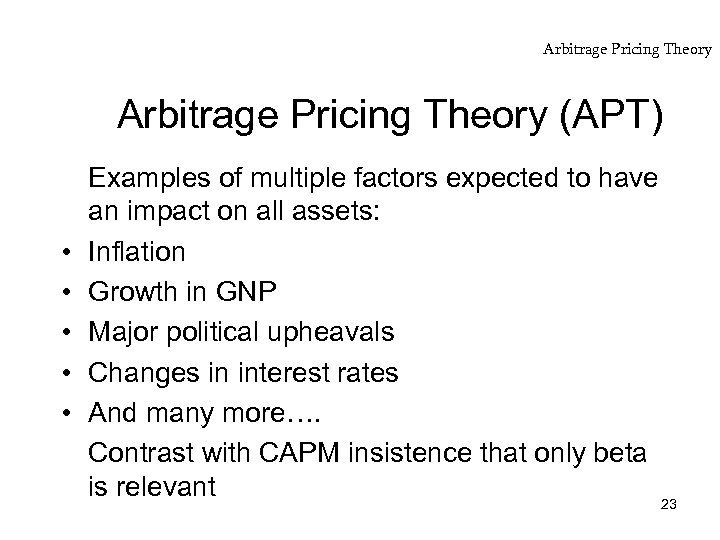Arbitrage Pricing Theory (APT) • • • Examples of multiple factors expected to have an impact on all assets: Inflation Growth in GNP Major political upheavals Changes in interest rates And many more…. Contrast with CAPM insistence that only beta is relevant 23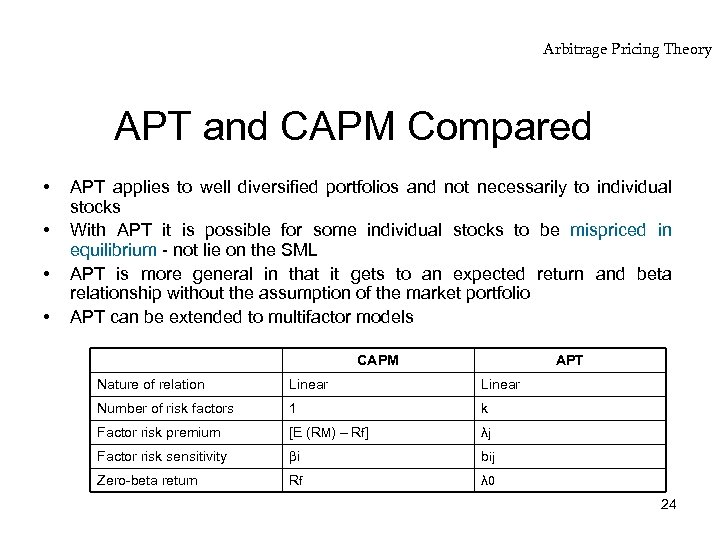Arbitrage Pricing Theory APT and CAPM Compared • • APT applies to well diversified portfolios and not necessarily to individual stocks With APT it is possible for some individual stocks to be mispriced in equilibrium - not lie on the SML APT is more general in that it gets to an expected return and beta relationship without the assumption of the market portfolio APT can be extended to multifactor models CAPM APT Nature of relation Linear Number of risk factors 1 k Factor risk premium [E (RM) – Rf] λj Factor risk sensitivity i bij Zero-beta return Rf λ 0 24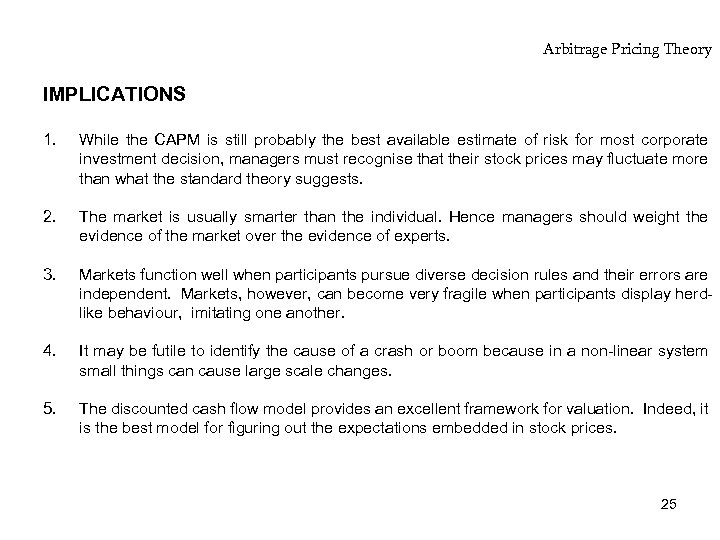Arbitrage Pricing Theory IMPLICATIONS 1. While the CAPM is still probably the best available estimate of risk for most corporate investment decision, managers must recognise that their stock prices may fluctuate more than what the standard theory suggests. 2. The market is usually smarter than the individual. Hence managers should weight the evidence of the market over the evidence of experts. 3. Markets function well when participants pursue diverse decision rules and their errors are independent. Markets, however, can become very fragile when participants display herdlike behaviour, imitating one another. 4. It may be futile to identify the cause of a crash or boom because in a non-linear system small things can cause large scale changes. 5. The discounted cash flow model provides an excellent framework for valuation. Indeed, it is the best model for figuring out the expectations embedded in stock prices. 25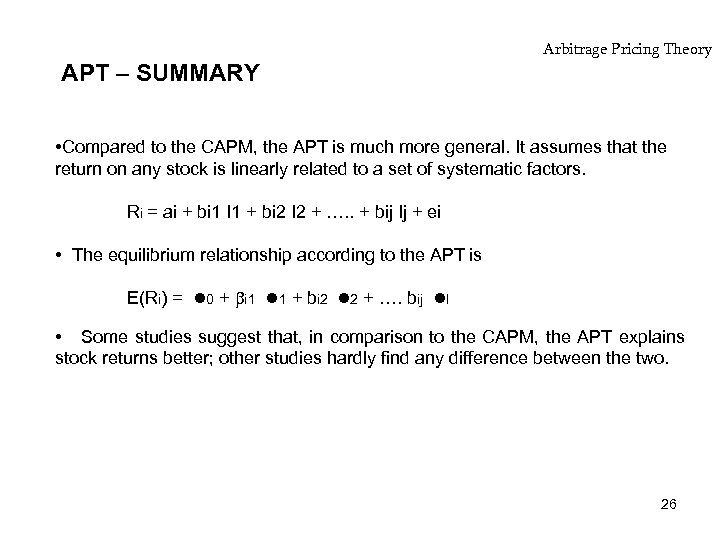APT – SUMMARY Arbitrage Pricing Theory • Compared to the CAPM, the APT is much more general. It assumes that the return on any stock is linearly related to a set of systematic factors. Ri = ai + bi 1 I 1 + bi 2 I 2 + …. . + bij Ij + ei • The equilibrium relationship according to the APT is E(Ri) = 0 + i 1 1 + bi 2 2 + …. bij I • Some studies suggest that, in comparison to the CAPM, the APT explains stock returns better; other studies hardly find any difference between the two. 26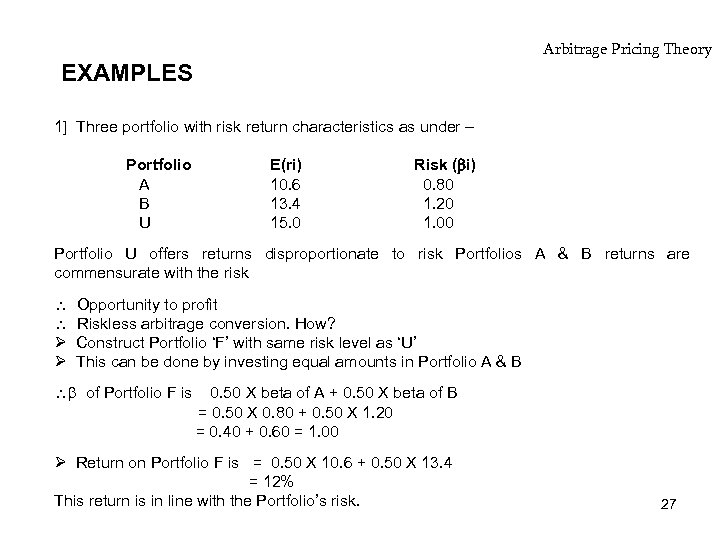Arbitrage Pricing Theory EXAMPLES 1] Three portfolio with risk return characteristics as under – Portfolio A B U E(ri) 10. 6 13. 4 15. 0 Risk ( i) 0. 80 1. 20 1. 00 Portfolio U offers returns disproportionate to risk Portfolios A & B returns are commensurate with the risk Ø Ø Opportunity to profit Riskless arbitrage conversion. How? Construct Portfolio ‘F’ with same risk level as ‘U’ This can be done by investing equal amounts in Portfolio A & B of Portfolio F is 0. 50 X beta of A + 0. 50 X beta of B = 0. 50 X 0. 80 + 0. 50 X 1. 20 = 0. 40 + 0. 60 = 1. 00 Ø Return on Portfolio F is = 0. 50 X 10. 6 + 0. 50 X 13. 4 = 12% This return is in line with the Portfolio’s risk. 27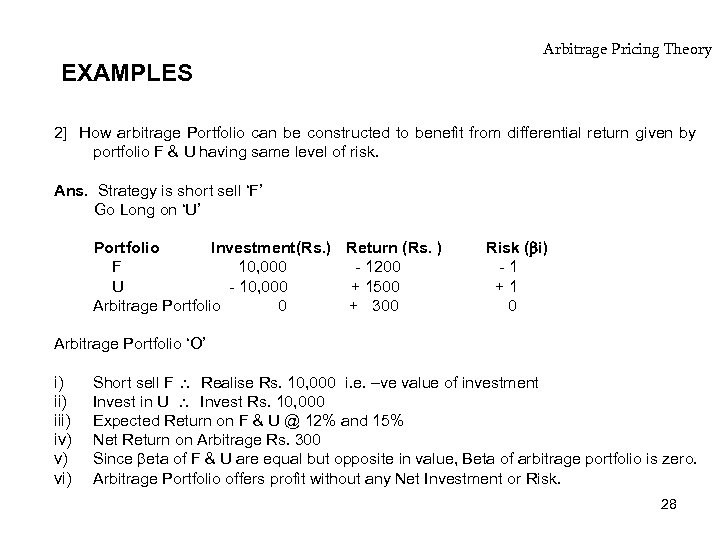EXAMPLES Arbitrage Pricing Theory 2] How arbitrage Portfolio can be constructed to benefit from differential return given by portfolio F & U having same level of risk. Ans. Strategy is short sell ‘F’ Go Long on ‘U’ Portfolio Investment(Rs. ) Return (Rs. ) F 10, 000 - 1200 U - 10, 000 + 1500 Arbitrage Portfolio 0 + 300 Risk ( i) -1 +1 0 Arbitrage Portfolio ‘O’ i) iii) iv) v) vi) Short sell F Realise Rs. 10, 000 i. e. –ve value of investment Invest in U Invest Rs. 10, 000 Expected Return on F & U @ 12% and 15% Net Return on Arbitrage Rs. 300 Since eta of F & U are equal but opposite in value, Beta of arbitrage portfolio is zero. Arbitrage Portfolio offers profit without any Net Investment or Risk. 28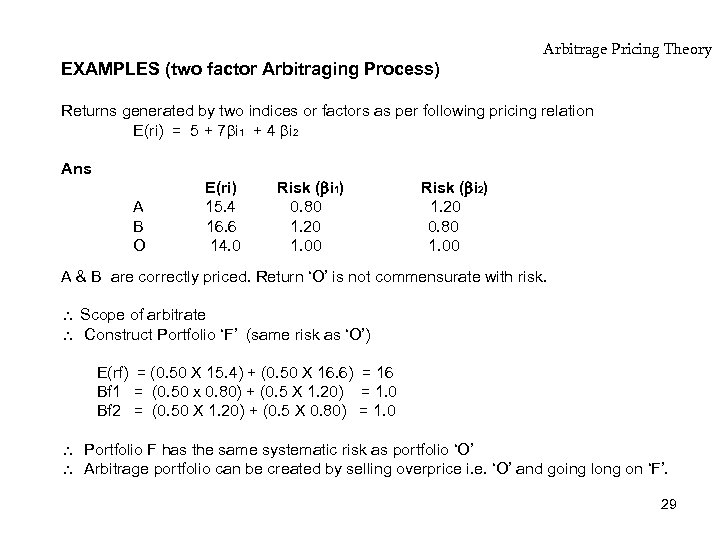EXAMPLES (two factor Arbitraging Process) Arbitrage Pricing Theory Returns generated by two indices or factors as per following pricing relation E(ri) = 5 + 7 i 1 + 4 i 2 Ans A B O E(ri) 15. 4 16. 6 14. 0 Risk ( i 1) 0. 80 1. 20 1. 00 Risk ( i 2) 1. 20 0. 80 1. 00 A & B are correctly priced. Return ‘O’ is not commensurate with risk. Scope of arbitrate Construct Portfolio ‘F’ (same risk as ‘O’) E(rf) = (0. 50 X 15. 4) + (0. 50 X 16. 6) = 16 Bf 1 = (0. 50 x 0. 80) + (0. 5 X 1. 20) = 1. 0 Bf 2 = (0. 50 X 1. 20) + (0. 5 X 0. 80) = 1. 0 Portfolio F has the same systematic risk as portfolio ‘O’ Arbitrage portfolio can be created by selling overprice i. e. ‘O’ and going long on ‘F’. 29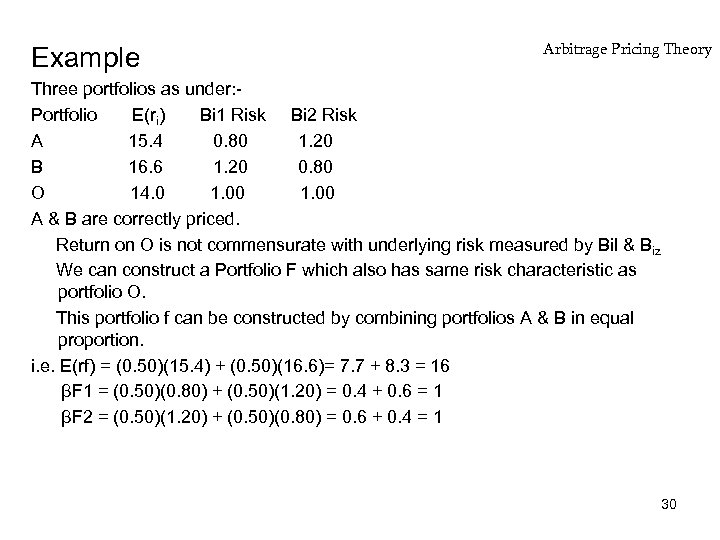Example Arbitrage Pricing Theory Three portfolios as under: Portfolio E(ri) Bi 1 Risk Bi 2 Risk A 15. 4 0. 80 1. 20 B 16. 6 1. 20 0. 80 O 14. 0 1. 00 A & B are correctly priced. Return on O is not commensurate with underlying risk measured by Bil & Biz We can construct a Portfolio F which also has same risk characteristic as portfolio O. This portfolio f can be constructed by combining portfolios A & B in equal proportion. i. e. E(rf) = (0. 50)(15. 4) + (0. 50)(16. 6)= 7. 7 + 8. 3 = 16 βF 1 = (0. 50)(0. 80) + (0. 50)(1. 20) = 0. 4 + 0. 6 = 1 βF 2 = (0. 50)(1. 20) + (0. 50)(0. 80) = 0. 6 + 0. 4 = 1 30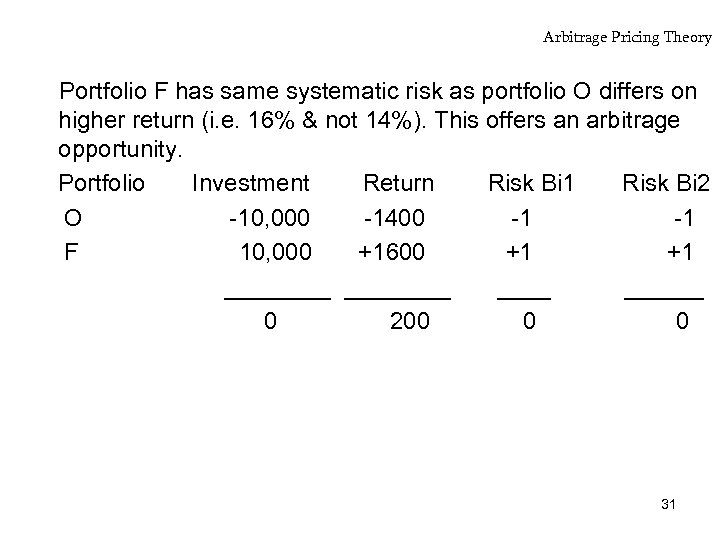Arbitrage Pricing Theory Portfolio F has same systematic risk as portfolio O differs on higher return (i. e. 16% & not 14%). This offers an arbitrage opportunity. Portfolio Investment Return Risk Bi 1 Risk Bi 2 O -10, 000 -1400 -1 -1 F 10, 000 +1600 +1 +1 ________ 0 200 0 0 31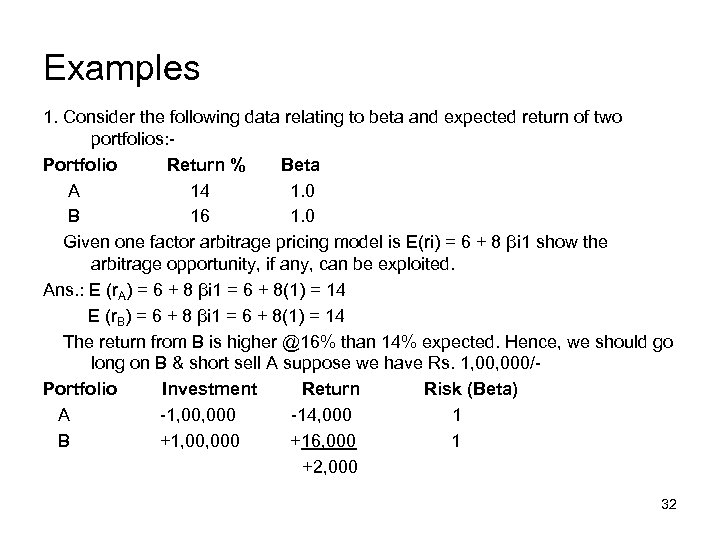Examples 1. Consider the following data relating to beta and expected return of two portfolios: Portfolio Return % Beta A 14 1. 0 B 16 1. 0 Given one factor arbitrage pricing model is E(ri) = 6 + 8 βi 1 show the arbitrage opportunity, if any, can be exploited. Ans. : E (r. A) = 6 + 8 βi 1 = 6 + 8(1) = 14 E (r. B) = 6 + 8 βi 1 = 6 + 8(1) = 14 The return from B is higher @16% than 14% expected. Hence, we should go long on B & short sell A suppose we have Rs. 1, 000/Portfolio Investment Return Risk (Beta) A -1, 000 -14, 000 1 B +1, 000 +16, 000 1 +2, 000 32